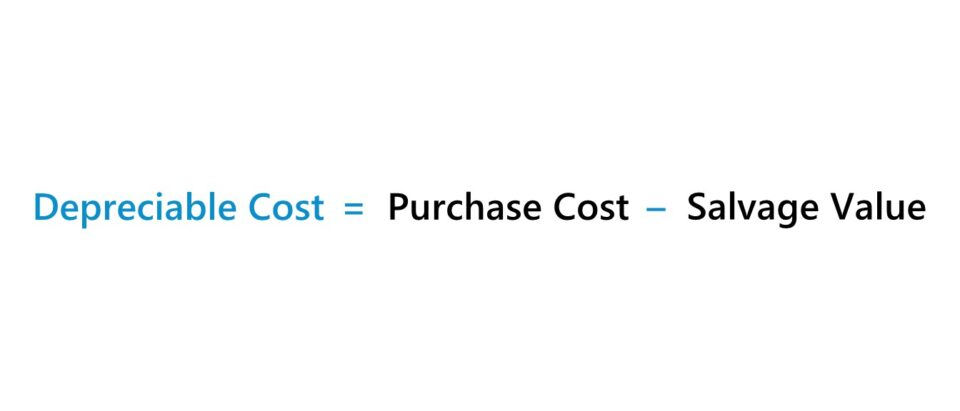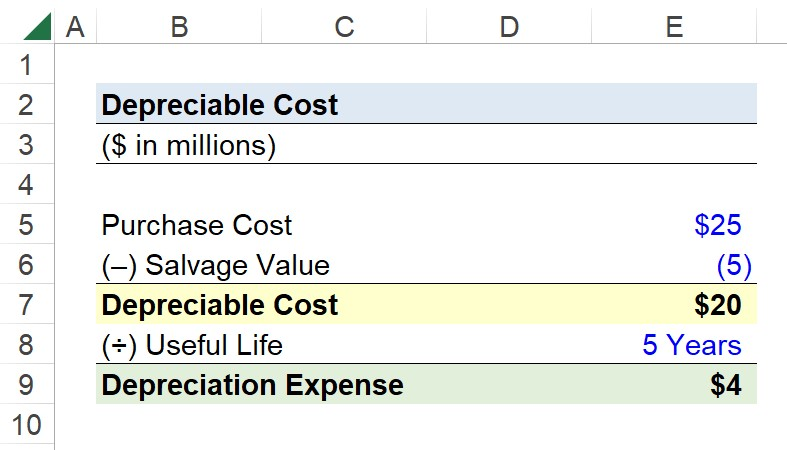Welcome to Wall Street Prep! Use code at checkout for 15% off.# Depreciable Cost

Guide to Understanding Depreciable Cost## How to Calculate Depreciable Cost?

The depreciable cost of a fixed asset represents the total value of the asset that can be depreciated over its useful life assumption.

The depreciable cost can be calculated as the purchase cost of the fixed asset minus its salvage value assumption.

• Purchase Cost → The total cost associated with the company acquiring the asset and completing the ownership transfer.
• Useful Life → The estimated number of periods in which the fixed asset is expected to provide positive economic utility.
• Salvage Value → The approximate value of the fixed asset remaining at the end of its useful life.

All else being equal, a higher depreciable cost coupled with a shorter useful life assumption and lower salvage value contribute towards a greater annual depreciation expense being recognized on the income statement.

## Depreciable Cost Formula

The formula to calculate the depreciable cost is as follows.

Depreciable Cost = Purchase Cost Salvage Value

Assuming the straight-line depreciation method (i.e. the equal allocation of the capital expenditure across its useful life), the depreciation expense is equal to the depreciable cost multiplied by the depreciation rate (%).

## Depreciable Cost Calculator – Excel Template

We’ll now move on to a modeling exercise, which you can access by filling out the form below.Submitting...

## Depreciable Cost Calculation Example

Suppose you’re tasked with calculating the depreciable cost of a fixed asset that was purchased for \$25 million.

The salvage value of the fixed asset is assumed to be \$5 million, while its useful life assumption is 5 years.

• Purchase Cost = \$25 million
• Salvage Value = \$5 million
• Useful Life = 5 Years

The depreciable cost is the difference between the purchase cost and salvage value, which comes out to \$20 million.

• Depreciable Cost = \$25 million – \$5 million = \$20 million

With the depreciable cost determined, we can divide it by the useful life assumption to arrive at an annual depreciation expense of \$4 million under the straight-line method.

• Depreciation Expense = \$20 million ÷ 5 Years = \$4 millionStep-by-Step Online Course

### Everything You Need To Master Financial Modeling

Enroll in The Premium Package: Learn Financial Statement Modeling, DCF, M&A, LBO and Comps. The same training program used at top investment banks.